# Geodesic geometry

(diff) ← Older revision | Latest revision (diff) | Newer revision → (diff)

geometry of geodesics

The geometry of a metric space (a-space) characterized by the fact that extensions of geodesic lines (cf. Geodesic line), defined as locally shortest lines, are unique.

A-space is defined by the following system of axioms:

1)is a metric space;is the distance in the space;

2)is finitely compact, i.e. bounded infinite sets inhave limit points;

3)is convex in the sense of Menger, i.e. for two points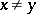there exists a third pointdistinct fromandand such that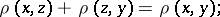4) for each pointthere exists an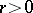such that in the ball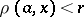there exists, for the points, a third pointdistinct fromandwith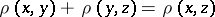(the axiom of local extension);

5) if, in axiom 4), two points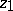and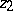such that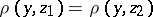have been found, then(the axiom of unique extension).

The class of-spaces includes, in particular, Riemannian spaces and Finsler spaces (cf. Finsler space; Riemannian space).-spaces in which extension of a geodesic is possible in general and any segment of the geodesic remains a shortest line are called straight spaces. They include, for example, Euclidean, Minkowski and Lobachevskii spaces, and all simply-connected Riemannian spaces of non-positive curvature. In straight spaces and in some-spaces of special type (elliptic), a geodesic is determined by two points.

In general-spaces, unlike in Minkowski spaces, a sphere is not always convex. Perpendicularity, defined in terms of the shortest line to the geodesic, is not necessarily symmetric, unlike in Euclidean spaces. Criteria have been formulated in terms of-spaces to distinguish Euclidean spaces, spheric spaces, and Minkowski spaces.

The theory of-spaces showed that many results of differential geometry are not connected with conditions of differentiability. This theory extended the studies on Finsler spaces; made it possible to study those metrizations of affine and projective spaces which convert straight lines to geodesic lines; and to study the freedom of choice of geodesic nets under metrization. A number of hitherto unsolved problems are connected with the possible topological structure of-spaces .

How to Cite This Entry:
Geodesic geometry. Encyclopedia of Mathematics. URL: http://encyclopediaofmath.org/index.php?title=Geodesic_geometry&oldid=32096
This article was adapted from an original article by V.A. Zalgaller (originator), which appeared in Encyclopedia of Mathematics - ISBN 1402006098. See original article